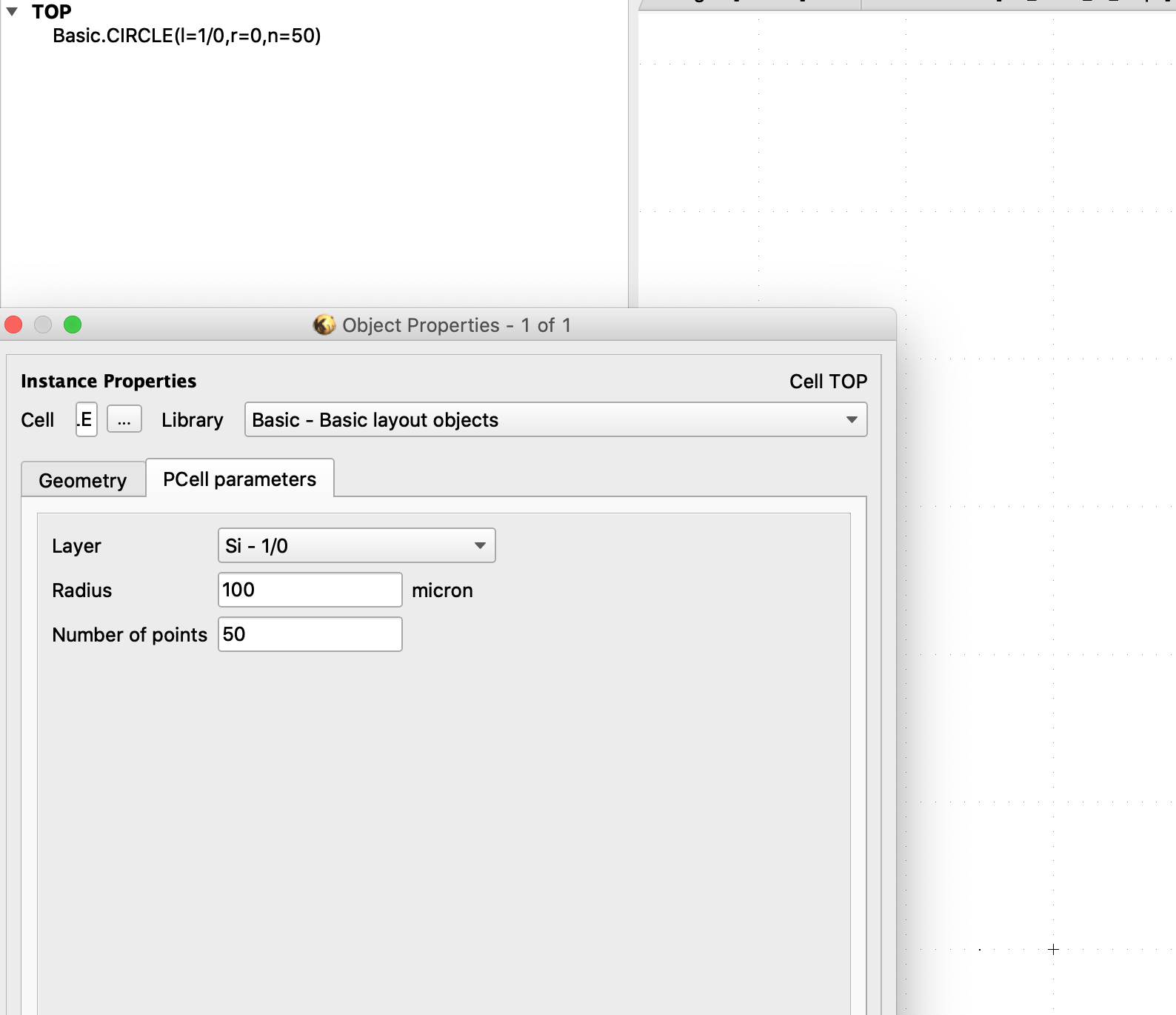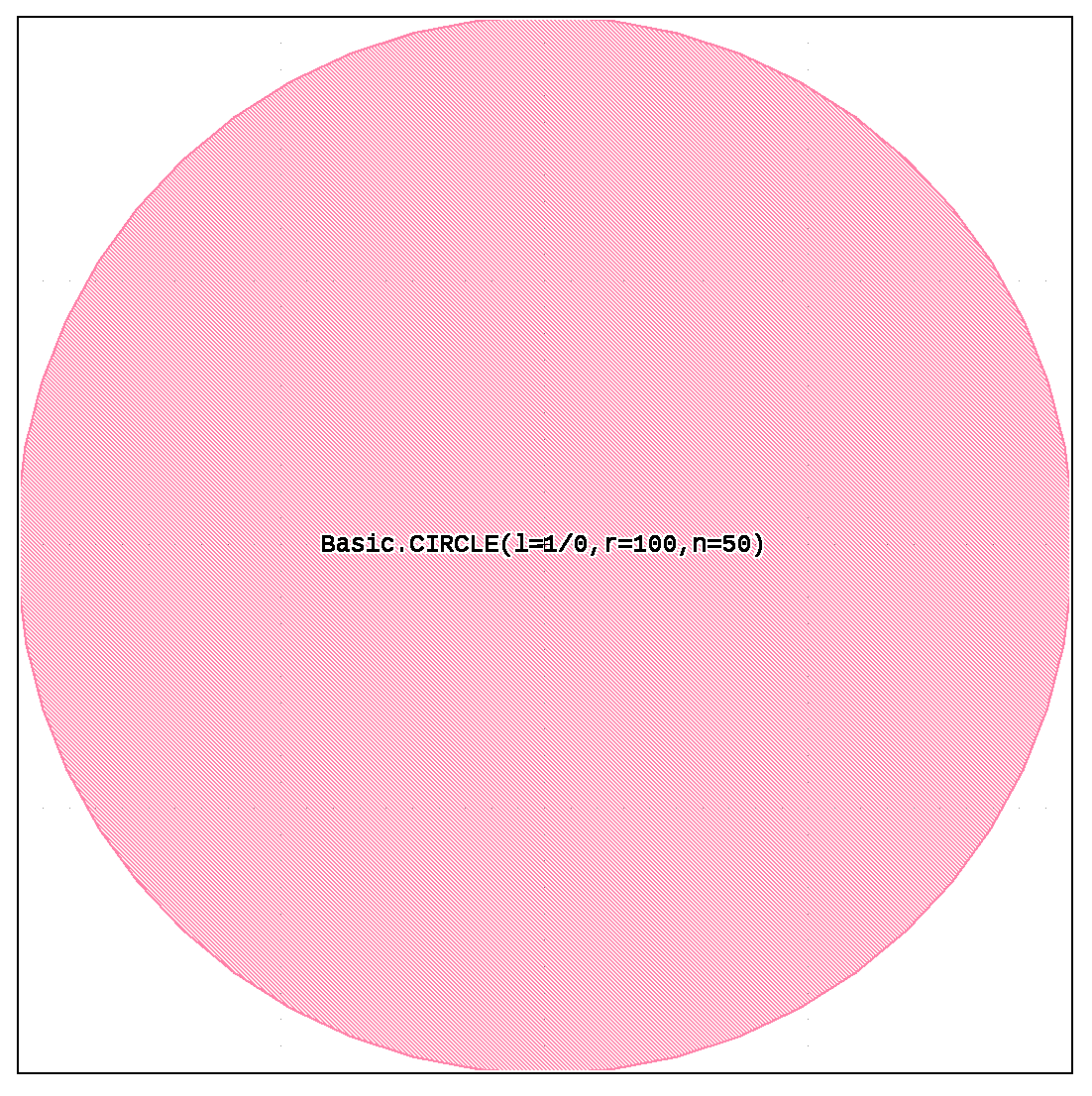# With Python BASIC TEXT pcell does not show up in layout

New to Python based KLayout, and trying to follow examples.

Why don't I see my p-cell in the layout? I want to instantiate the BASIC cell with some text. The code is shown below:

``````import pya
pya.MainWindow.instance().create_layout(0)
view = pya.LayoutView.current()
L = pya.CellView.active().layout()

txt_layer=L.layer(1,0)

topcell = L.create_cell("TOP")
param   = {"layer":txt_layer,"text":lum_lib_txt,  "mag":10}

txtcell = L.create_cell("TEXT", "Basic", param)
trans=pya.Trans.new(0,False,0,0)

lib = pya.Library.library_by_name("Basic")
pcell_decl = lib.layout().pcell_declaration("TEXT")

param   = {"text":"foo", "layer":txt_layer, "mag":10}

pv = []
for p in pcell_decl.get_parameters():
if p.name in param:
pv.append(param[p.name])
else:
pv.append(p.default)

topcell.insert(pya.CellInstArray.new(txtcell.cell_index(),trans))
``````

• The code has a couple of flaws.

The basic problem is to properly create the layers and make them visible. Here is some working code:

``````import pya

lum_lib_txt = "HELLO, WORLD!"

pya.MainWindow.instance().create_layout(0)

view = pya.LayoutView.current()
cv = pya.CellView.active()

L = cv.layout()

# the layer where to put the text to
txt_layer = pya.LayerInfo(1, 0)

# create layer 1/0
L.layer(txt_layer)

topcell = L.create_cell("TOP")
param  = { "layer": txt_layer, "text": lum_lib_txt, "mag": 10 }

# create the PCell variant
txtcell = L.create_cell("TEXT", "Basic", param)

# insert the PCell variant into the top cell
trans = pya.Trans(0, False, 0, 0)
topcell.insert(pya.CellInstArray.new(txtcell.cell_index(), trans))

# make the new layer visible

# Make the top cell the current one
cv.cell = topcell

# zoom
view.zoom_fit()
``````

Matthias

• Hi Matthias,

I modified your code for a Basic.CIRCLE, and found un-expected behaviour.

After running, the cell shows up as "Basic.CIRCLE(l=1/0,r=0,n=64)" and I don't see the layout:Code:

``````import pya

pya.MainWindow.instance().create_layout(0)

view = pya.LayoutView.current()
cv = pya.CellView.active()

L = cv.layout()

# the layer where to put the text to
txt_layer = pya.LayerInfo(1, 0)

# create layer 1/0
L.layer(txt_layer)

topcell = L.create_cell("TOP")
#param  = { "layer": txt_layer, "text": lum_lib_txt, "mag": 10 }
param = {"layer": txt_layer , "radius": 100, "npoints": 64}

# create the PCell variant
ccell = L.create_cell("CIRCLE", "Basic", param)

# insert the PCell variant into the top cell
trans = pya.Trans(0, False, 0, 0)
topcell.insert(pya.CellInstArray.new(ccell.cell_index(), trans))

# make the new layer visible

# Make the top cell the current one
cv.cell = topcell

# zoom
view.zoom_fit()
``````

Then I check the parameters

``````print(ccell.pcell_parameters())
``````

and get the correct ones:

``````[<pya.LayerInfo object at 0x7fb7aa1c4560>, 100, <pya.DPoint object at 0x7fb7ac37add0>, 50, 0.0]
``````

Then I edit the cell Properties, and click OK, and the cell updates itself and draws correctly.• edited December 2020

For the Circle from Basic.CIRCLE, the radius to use for programmatic instantiation is "actual_radius", not "radius". This PCell has two ways of setting the radius: on through the entry box and by moving the handle. "actual_radius" is the one that is taken for computing the circle and it's the consolidated version of both input channels.

But if it's just about creating circles, use "Polygon.ellipse(bounding_box)". It's roughly a hundred times faster and leaner.

Matthias

• Thank you.

• Hello Matthias,

I did not realize you had responded. Thanks so much for your help.